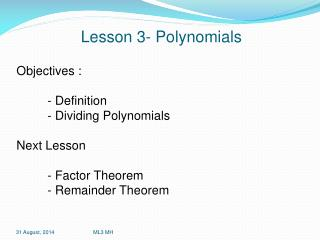DownloadDownload PresentationLesson 3- Polynomials

# Lesson 3- Polynomials

Télécharger la présentation## Lesson 3- Polynomials

- - - - - - - - - - - - - - - - - - - - - - - - - - - E N D - - - - - - - - - - - - - - - - - - - - - - - - - - -
##### Presentation Transcript

1. Lesson 3- Polynomials Objectives : - Definition - Dividing Polynomials Next Lesson - Factor Theorem - Remainder Theorem ML3 MH

2. Polynomial Real numbers called coefficients Constant n is the Degree of the polynomial ML3 MH

3. Multiplying Polynomials • Expand all the terms ML3 MH

4. Dividing Polynomials • This is trickier than multiplication • There are two main ways • Long Division • By Inspection ML3 MH

6. Algebraic long division Divide 2x³ + 3x² - x + 1 by x + 2 x + 2 is the divisor 2x³ + 3x² - x + 1 is the dividend The quotient will be here. ML3 MH

7. Algebraic long division First divide the first term of the dividend, 2x³, by x (the first term of the divisor). This gives 2x². This will be the first term of the quotient. ML3 MH

8. Algebraic long division Now multiply 2x² by x + 2 and subtract ML3 MH

9. Algebraic long division Bring down the next term, -x. ML3 MH

10. Algebraic long division Now divide –x², the first term of –x² - x, by x, the first term of the divisor which gives –x. ML3 MH

11. Algebraic long division Multiply –x by x + 2 and subtract ML3 MH

12. Algebraic long division Bring down the next term, 1 ML3 MH

13. Algebraic long division Divide x, the first term of x + 1, by x, the first term of the divisor which gives 1 ML3 MH

14. Algebraic long division Multiply x + 2 by 1 and subtract ML3 MH

15. Algebraic long division The quotient is 2x² - x + 1 The remainder is –1. ML3 MH

17. Dividing in your head Divide 2x³ + 3x² - x + 1 by x + 2 When a cubic is divided by a linear expression, the quotient is a quadratic and the remainder, if any, is a constant. Let the quotient by ax² + bx + c Let the remainder be d. 2x³ + 3x² - x + 1 = (x + 2)(ax² + bx + c) + d ML3 MH

18. Dividing in your head The first terms in each bracket give the term in x³ 2x³ + 3x² - x + 1 = (x + 2)(ax² + bx + c) + d xmultiplied by ax² gives ax³ so a must be 2. ML3 MH

19. Dividing in your head The first terms in each bracket give the term in x³ 2x³ + 3x² - x + 1 = (x + 2)(2x² + bx + c) + d x multiplied by ax² gives ax³ so a must be 2. ML3 MH

20. Dividing in your head Now look for pairs of terms that multiply to give terms in x² 2x³ + 3x² - x + 1 = (x + 2)(2x² + bx + c) + d x multiplied by bx gives bx² 2 multiplied by 2x² gives 4x² bx² + 4x² must be 3x² so b must be -1. ML3 MH

21. Dividing in your head Now look for pairs of terms that multiply to give terms in x² 2x³ + 3x² - x + 1 = (x + 2)(2x² + -1x + c) + d x multiplied by bx gives bx² 2 multiplied by 2x² gives 4x² bx² + 4x² must be 3x² so b must be -1. ML3 MH

22. Dividing in your head Now look for pairs of terms that multiply to give terms in x 2x³ + 3x² - x + 1 = (x + 2)(2x² - x + c) + d x multiplied by c gives cx 2 multiplied by -x gives -2x cx - 2x must be -x so c must be 1. ML3 MH

23. Dividing in your head Now look for pairs of terms that multiply to give terms in x 2x³ + 3x² - x + 1 = (x + 2)(2x² - x + 1) + d x multiplied by c gives cx 2 multiplied by -x gives -2x cx - 2x must be -x so c must be 1. ML3 MH

24. Dividing in your head Now look at the constant term 2x³ + 3x² - x + 1 = (x + 2)(2x² - x + 1) + d 2 multiplied by 1 gives 2 then add d 2 + d must be 1 so d must be -1. ML3 MH

25. Dividing in your head Now look at the constant term 2x³ + 3x² - x + 1 = (x + 2)(2x² - x + 1) - 1 2 multiplied by 1 gives 2 then add d 2 + d must be 1 so d must be -1. ML3 MH

26. Dividing in your head 2x³ + 3x² - x + 1 = (x + 2)(2x² - x + 1) - 1 The quotient is 2x² - x + 1 and the remainder is –1. ML3 MH